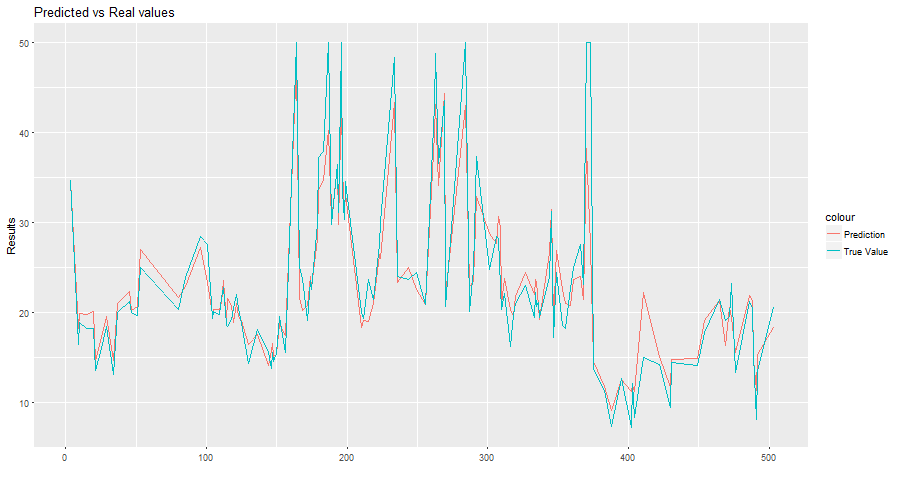# Train Locally and Predict in Exasol

This section describes how you can train your data locally and predict in Exasol. Start by loading the Boston Housing dataset in R. The dataset is available through the `mlbench` package.

``# load librarieslibrary(mlbench)         # Load datalibrary(RODBC)           # ODBC database connectivitylibrary(exasol)          # R interface for Exasol databaselibrary(randomForest)    # Random forests for regression and classificationlibrary(RCurl)           # HTTP communication``

After loading the data in your environment, make a split for training and testing sets. There are many methods to it, one of them is to randomly generate indexes that will subset rows from the main set.

The ratio of the split is arbitrary and depends on available data and application. In this case, keep around 75% of the data for training and the remaining 25% for testing purpose.

``# Load datadata("BostonHousing")# Generate random indices (75% of the sample size)set.seed(42)indices <- sample(nrow(BostonHousing),size=floor(nrow(BostonHousing) * 0.75))# Add a dummy variable with 2 levels "Train" and "Test"BostonHousing\$split = factor(NA, levels = c("Train", "Test"))# Assign predefined rows respectively to "Train" and "Test" subsetsBostonHousing\$split[indices]  = "Train"BostonHousing\$split[-indices] = "Test"# Add id variableBostonHousing\$id = seq(1:nrow(BostonHousing))``

Now you can train a basic random forest model on our data and see which variables contribute the most to predicting the median house value.

``set.seed(42)# Run model (note that we filter only for the training data (split=="Train"))rf_model = randomForest(medv ~ .-split -id,                        data = BostonHousing[BostonHousing\$split=="Train",],                        importance = TRUE)# See which variables are important in predicting house pricesvarImpPlot(rf_model)# rm    - number of rooms per house# lstat - % lower status of the population``## Send model to BucketFS

UDF scripts are executed in parallel on the Exasol cluster and in this case for the prediction such script needs to have access to the model we just trained. It's possible to use any file service, however when considering performance, it is more efficient to have a storage space in the local cluster nodes.

Keep in mind that the Exasol database cluster stores only tables and you cannot upload our model in there.

Exasol BucketFS file system has been developed for such use cases, where data should be stored synchronously and replicated across the cluster. This concept can be used to extend script languages and even to install completely new script languages on the Exasol cluster. For more information, see Adding New Packages to Existing Script Languages.

Files can be sent and retrieved from BucketFS in the following ways:

• Using packages that allow HTTP communication in R (`httr` or `RCurl`)
• Using `curl` commands (not covered here)

With the first method we will use the `RCurl` package. It is important to note that the model which lives in our R environment now has to be serialized (converted into raw text). When we want to retrieve it we can unserialize it and return it to its original file type. See `?serialize` for more information.

``# Define options for the authenticationcurl_opts = curlOptions(userpwd  = "w:<write_passwd>",                        verbose  = FALSE,                        httpauth = AUTH_BASIC)# Transfer model to the buckethttpPUT(  # EXABucket URL (change for your environment)  url = "http://<Exasol server>:<BucketFS service port>/<Bucket name>/rf_model",  # It is important to serialize the model  content = serialize(rf_model, ascii = FALSE, connection = NULL),  # EXABucket: authenticate  curl = getCurlHandle(.opts = curl_opts))``

If the size of you model increases, you can use the Strip package that allows you to reduce the memory footprint of your models.

### Send data to Exasol

To run the prediction in Exasol you need to transfer the data into the database. To know how to write and read data into Exasol, see Write Data and Read Data.

``# Create connection with the Exasol databaseexaconn <- dbConnect(    drv     = "exa",                  # EXAdriver object    exahost = "192.168.56.103:8563",  # IP of database cluster    uid     = "sys",                  # Username    pwd     = "exasol")               # Password# Create database schema (if it does not yet exist) with the name r_demo# (This also opens it, i.e. makes it the default container for all subsequent steps below)odbcQuery(exaconn, "CREATE SCHEMA IF NOT EXISTS r_demo")# If the schema already existed, we need to open it to make it the default container for all subsequent steps belowodbcQuery(exaconn, "OPEN SCHEMA r_demo")# Create an empty table in Exasol with the name boston_housingodbcQuery(exaconn,    "CREATE OR REPLACE TABLE boston_housing(         crim    DOUBLE,         zn      DOUBLE,         indus   DOUBLE,         chas    VARCHAR(10),         nox     DOUBLE,         rm      DOUBLE,         age     DOUBLE,         dis     DOUBLE,         rad     DOUBLE,         tax     DOUBLE,         ptratio DOUBLE,         b       DOUBLE,         lstat   DOUBLE,         medv    DOUBLE,         split   VARCHAR(10),         id      INT     )")# Write the train data into Exasolexa.writeData(exaconn, data = BostonHousing, tableName = "boston_housing")``

Through an SQL client, you can see the data that is transferred to the database with the corresponding schema.### Make prediction using UDF

You are now ready to use the algorithm which is in BucketFS to run a prediction on the data that's in Exasol. For this, you can create a UDF using the `exa.createScript` function.

``PredictInExasol1 <- exa.createScript(  exaconn,  "r_demo.dt_predict1",  function(data) {    # Load the required packages    require(RCurl)    require(randomForest)    # Load data in chuks of 1000 rows at a time (very useful knob with big data!)    repeat {      if (!data\$next_row(1000))        break      # put data in a data.frame      df <- data.frame(              id       = data\$id,              crim     = data\$crim,              zn       = data\$zn,              indus    = data\$indus,              chas     = data\$chas,              nox      = data\$nox,              rm       = data\$rm,              age      = data\$age,              dis      = data\$dis,              rad      = data\$rad,              tax      = data\$tax,              ptratio  = data\$ptratio,              b        = data\$b,              lstat    = data\$lstat,              medv     = data\$medv,              split    = data\$split            )    }    # Set options for retrieving model from bucket    curl_opts = curlOptions(userpwd = "w:<write_passwd>",                            verbose = TRUE,                            httpauth=AUTH_BASIC)    # Loading the model from the bucket (note that is unserialized)    # Change the bucket information for your environment    rf_model = unserialize(file("/buckets/<BucketFS service name>/<Bucket name>/rf_model", "rb"))    # Use the loaded model to make the prediction    prediction <- predict(rf_model, newdata = df)    # Return of the forecast    data\$emit(df\$id, df\$medv, prediction)  },  # Input arguments  inArgs  = c("id         INT",              "crim       DOUBLE",              "zn         DOUBLE",              "indus      DOUBLE",              "chas       VARCHAR(10)",              "nox        DOUBLE",              "rm         DOUBLE",              "age        DOUBLE",              "dis        DOUBLE",              "rad        DOUBLE",              "tax        DOUBLE",              "ptratio    DOUBLE",              "b          DOUBLE",              "lstat      DOUBLE",              "medv       DOUBLE",              "split      VARCHAR(10)"),  # Output arguments  outArgs = c("id         INT",              "RealValue  DOUBLE",              "Prediction DOUBLE"))# Create a table with the real values and predicted ones# Note that the prediction is done in test data using 'where' argumentprediction_output = PredictInExasol1("id","crim", "zn", "indus", "chas", "nox", "rm", "age", "dis",                                     "rad", "tax", "ptratio", "b", "lstat", "medv", "split",                                     table = "r_demo.boston_housing",                                     groupBy = "iproc(),mod(iproc(),5)",                                     where = "split = 'Test'")# Check the root mean squared error (RMSE)RMSE = sqrt(mean((prediction_output\$PREDICTION - prediction_output\$REALVALUE)^2))# Should be 3.35``

You can also plot your predicted values and see the output:

``# Plot the predictions against real valueslibrary(ggplot2)plot_predictions = ggplot(prediction_output, aes(ID)) +                    geom_line(aes(y = PREDICTION, colour = "Prediction")) +                    geom_line(aes(y = REALVALUE, colour = "True Value")) +                    xlab("") +                    ylab("Results") +                    ggtitle("Predicted vs Real values")                    theme(legend.title=element_blank())plot(plot_predictions)``